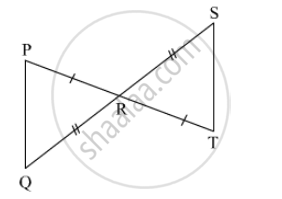SSC (English Medium) Class 8Maharashtra State Board
Share

# In Each Pair of Triangles Given Below, Parts Shown by Identical Marks Are Congruent. State the Test and the One to One Correspondence of Vertices by Which Triangles in Each Pair Are Congruent - SSC (English Medium) Class 8 - Mathematics

ConceptCongruence of Triangles

#### Question

In the triangle given below, parts shown by identical marks are congruent. State the test and the one to one correspondence of vertices by which triangles in each pair are congruent and remaining congruent parts.#### SolutionIn ∆PRQ and ∆TRS,
side PR ≅ side RT (given)
side QR ≅ side RS (given)
∠PRQ ≅ ∠SRT (vertically opposite angles)
∴ By SAS test, ∆PRQ ≅ ∆TRS.
∴ side PQ ≅ side ST,
∠QPR ≅ ∠STR,
∠PQR ≅ ∠TSR.

Is there an error in this question or solution?

#### APPEARS IN

Balbharati Solution for Balbharati Class 8 Mathematics (2019 to Current)
Chapter 13: Congruence of triangles
Practice Set 13.2 | Q: 1.2 | Page no. 87
Solution In Each Pair of Triangles Given Below, Parts Shown by Identical Marks Are Congruent. State the Test and the One to One Correspondence of Vertices by Which Triangles in Each Pair Are Congruent Concept: Congruence of Triangles.
S